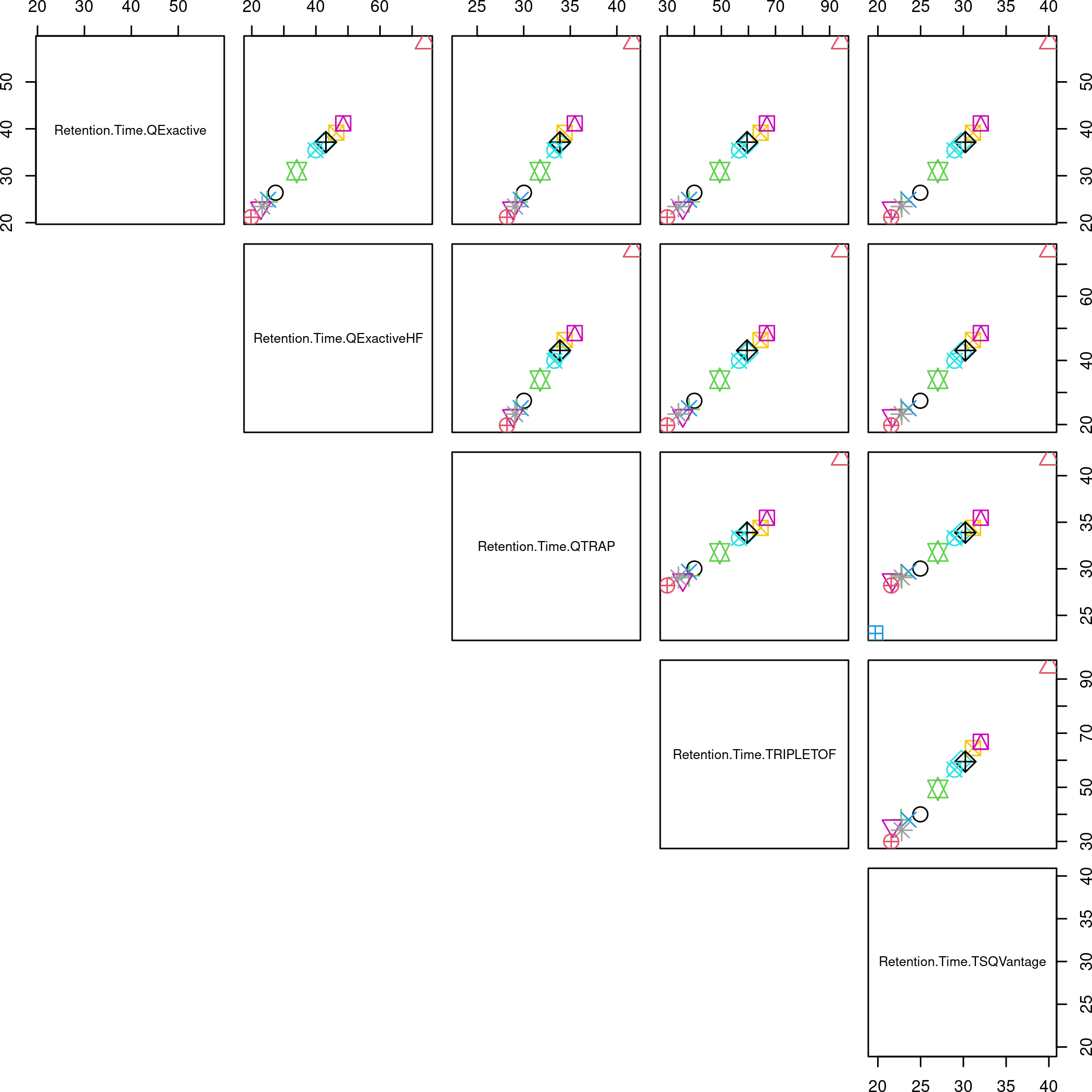# 1 Introduction

This vignette file is thought to demonstrate the retention time stability of the `msqc1_8rep` and `msqc1_dil` data contained in the `msqc1` R package.

## 1.1 Problem:

Retention Time (RT) form different LC-MS platforms are often not comparable (duration, offset).

## 1.2 Solution: Normalization

The iRT concept [proposed in pmid22577012 for the RT normalization is used. Instead of using the iRT-peptides MSQC1-peptides are used as reference peptides between the different chromatographic settings and systems.

The normalization is done by applying the following steps:

• define a reference platform (QTRAP)
• for each platform a linear model is build (in the following we use the `stats::lm` function) which maps the RT space of the corresponding platform into reference platform RT space (by using the `stats::predict.lm` method)
• scale the normalized RT values into [0, 1]
• visualize the normalized RT values

The code below shows the used R functions for the applied RT normalization.

``msqc1:::.normalize_rt``
``````## function (S, S.training, reference_instrument = "Retention.Time.QTRQP")
## {
##     S.normalized <- S
##     for (instrument in unique(S.normalized\$instrument)) {
##         i <- paste("Retention.Time", instrument, sep = ".")
##         rt.out <- S.training[, reference_instrument]
##         rt.in <- S.training[, i]
##         S.fit <- lm(rt.out ~ rt.in)
##         S.normalized[S.normalized\$instrument == instrument, "Retention.Time"] <- predict(S.fit,
##             data.frame(rt.in = S.normalized[S.normalized\$instrument ==
##                 instrument, "Retention.Time"]))
##     }
##     S.normalized.min <- min(S.normalized\$Retention.Time, na.rm = TRUE)
##     S.normalized.delta <- max(S.normalized\$Retention.Time, na.rm = TRUE) -
##         S.normalized.min
##     S.normalized\$Retention.Time <- (S.normalized\$Retention.Time -
##         S.normalized.min)/S.normalized.delta
##     return(S.normalized)
## }
## <environment: namespace:msqc1>``````

The `reshape_rt` method is used for reshaping the data from long to wide format which ease the the model building in the `msqc1:::.normalize_rt` method above.

``msqc1:::.reshape_rt``
``````## function (S, peptides = peptides, plot = TRUE, ...)
## {
##     S <- S[grep("[by]", S\$Fragment.Ion), ]
##     S <- S[S\$Peptide.Sequence %in% peptides\$Peptide.Sequence,
##         ]
##     S <- aggregate(Retention.Time ~ Peptide.Sequence * instrument,
##         FUN = mean, data = S)
##     S <- droplevels(S)
##     S.training <- reshape(S, direction = "wide", v.names = "Retention.Time",
##         timevar = c("instrument"), idvar = "Peptide.Sequence")
##     if (plot == TRUE) {
##         pairs(S.training[, 2:6], pch = as.integer(S.training\$Peptide.Sequence),
##             col = as.integer(S.training\$Peptide.Sequence), lower.panel = NULL,
##             ...)
##     }
##     return(S.training)
## }
## <bytecode: 0x563e27994658>
## <environment: namespace:msqc1>``````

The following R code listings displays some helper functions designed to ease the visualization of the `msqc1_8rep` and `msqc1_dil` RT values.

``msqc1:::.plot_rt_8rep``
``````## function (S, peptides, ...)
## {
##     .figure_setup()
##     S <- S[grep("[by]", S\$Fragment.Ion), ]
##     S <- S[S\$Peptide.Sequence %in% peptides\$Peptide.Sequence,
##         ]
##     S <- aggregate(Retention.Time ~ Peptide.Sequence * File.Name.Id *
##         instrument * relative.amount * Isotope.Label.Type, FUN = mean,
##         data = S)
##     S <- droplevels(S)
##     xyplot(Retention.Time ~ File.Name.Id | Isotope.Label.Type *
##         instrument, data = S, layout = c(10, 1), group = S\$Peptide.Sequence,
##         auto.key = list(space = "right", points = TRUE, lines = FALSE,
##             cex = 1), ...)
## }
## <bytecode: 0x563e29e8bde0>
## <environment: namespace:msqc1>``````
``msqc1:::.plot_rt_dil``
``````## function (S, peptides, ...)
## {
##     .figure_setup()
##     S <- S[grep("[by]", S\$Fragment.Ion), ]
##     S <- S[S\$Peptide.Sequence %in% peptides\$Peptide.Sequence,
##         ]
##     S <- aggregate(Retention.Time ~ Peptide.Sequence * File.Name *
##         instrument * relative.amount * Isotope.Label.Type, FUN = mean,
##         data = S)
##     S <- droplevels(S)
##     xyplot(Retention.Time ~ relative.amount | Isotope.Label.Type *
##         instrument, data = S, layout = c(10, 1), group = S\$Peptide.Sequence,
##         scales = list(x = list(rot = 45, log = TRUE, at = sort(unique(S\$relative.amount)))),
##         auto.key = list(space = "right", points = TRUE, lines = FALSE,
##             cex = 1), ...)
## }
## <bytecode: 0x563e29dcccc0>
## <environment: namespace:msqc1>``````

# 2 RT plot

## 2.1 8 Technical Replicates RT plot

Prepare the training data for the linear model.

``S.training.8rep <- msqc1:::.reshape_rt(msqc1_8rep, peptides=peptides, cex=2)``8 rep Scatterplot Matrices Plot - Color and icon type indicates the differnet peptides. On an idea plot all values would be on one line.

``msqc1:::.plot_rt_8rep(msqc1_8rep, peptides=peptides, xlab='Replicate Id')``